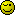# Php array_sum not working properly

Hello there!

I’m having a strange problem. I’m trying to get an average of numbers from a table.
I created an array, then imploded it but when I give the value, it only outputs the first number not the average I need.
What am I doing wrong?

``````       <?php
(...)
foreach(\$lr_loop as \$row):
\$loc_rate[] = \$row["stars"];
endforeach;
\$loc_rate_imploded = implode(', ', \$loc_rate);

echo \$loc_rate_imploded; // Gives 5,2,3,5,4 etc.
\$array = array(\$loc_rate_imploded);
echo "<br><br>";
\$avg = array_sum(\$array) / count(\$array);

echo \$avg; //Only shows the first number, in this case: 5
?>
``````

Just out of interest, what do you get if you `var_dump` \$array?

This is interesting…

``````array(1) { => string(10) "3, 5, 2, 5" }
``````

\$loc_rate is the array of values you should be using.

Putting the imploded string into a php array() creates an array of one element consisting of that string. While that produces somethings that ‘looks’ like the syntax definition for creating an array, it’s not because the string of values isn’t php code.

You mean like this?

``````       <?php
foreach(\$lr_loop as \$row):
\$loc_rate[] = \$row["stars"];
endforeach;

\$array = array(\$loc_rate);
echo "<br><br>";
\$avg = array_sum(\$array) / count(\$array);

echo \$avg;

?>

``````

It now outputs 0.

``````array(1) { => array(4) { => string(1) "3" => string(1) "5" => string(1) "2" => string(1) "5" } }
``````

No. Why (Jeopardy theme playing in the background) are you storing the fetched data in the \$loc_rate array if you are not going to use that array?

1 Like

Ok, I’m feeling a bit stupid now…Thanks by the way``````\$array = array_values(\$loc_rate);
``````

I think the point that @mabismad was making is, you already have an array of all those values, you build it in your `foreach()` loop right at the start. So why do you need to copy it into `\$array`? (BTW - terrible name for an array).

This line

``````\$array = array_values(\$loc_rate);
``````

doesn’t really add anything, because (unless your code is a simplified version and there’s more to the full version) your original `\$locrate` array doesn’t have any keys to start with.

Also, this:

``````foreach(\$lr_loop as \$row):
\$loc_rate[] = \$row["stars"];
endforeach;
``````

can be written as:

``````\$loc_rate = array_column(\$lr_loop, 'stars');
``````

This topic was automatically closed 91 days after the last reply. New replies are no longer allowed.